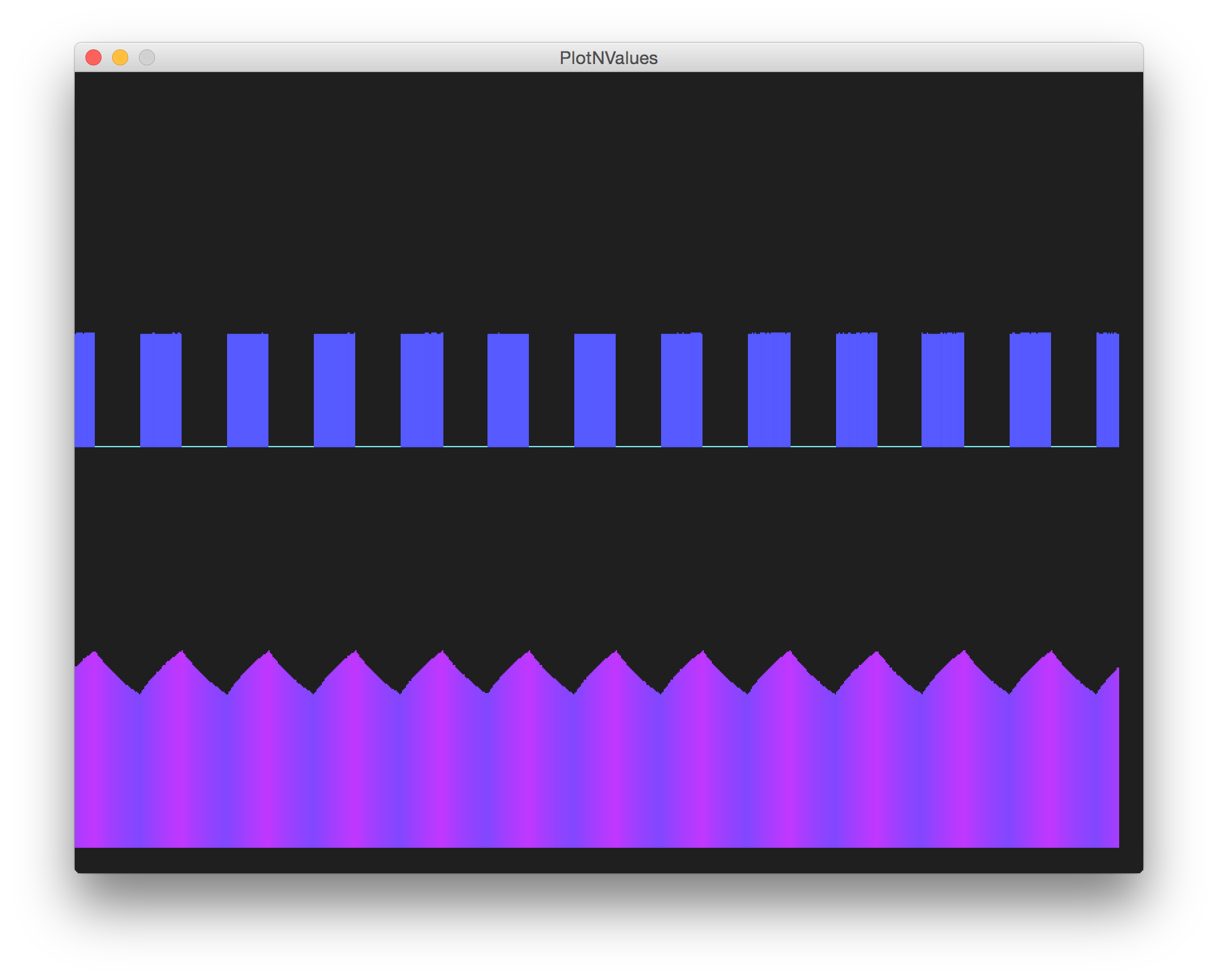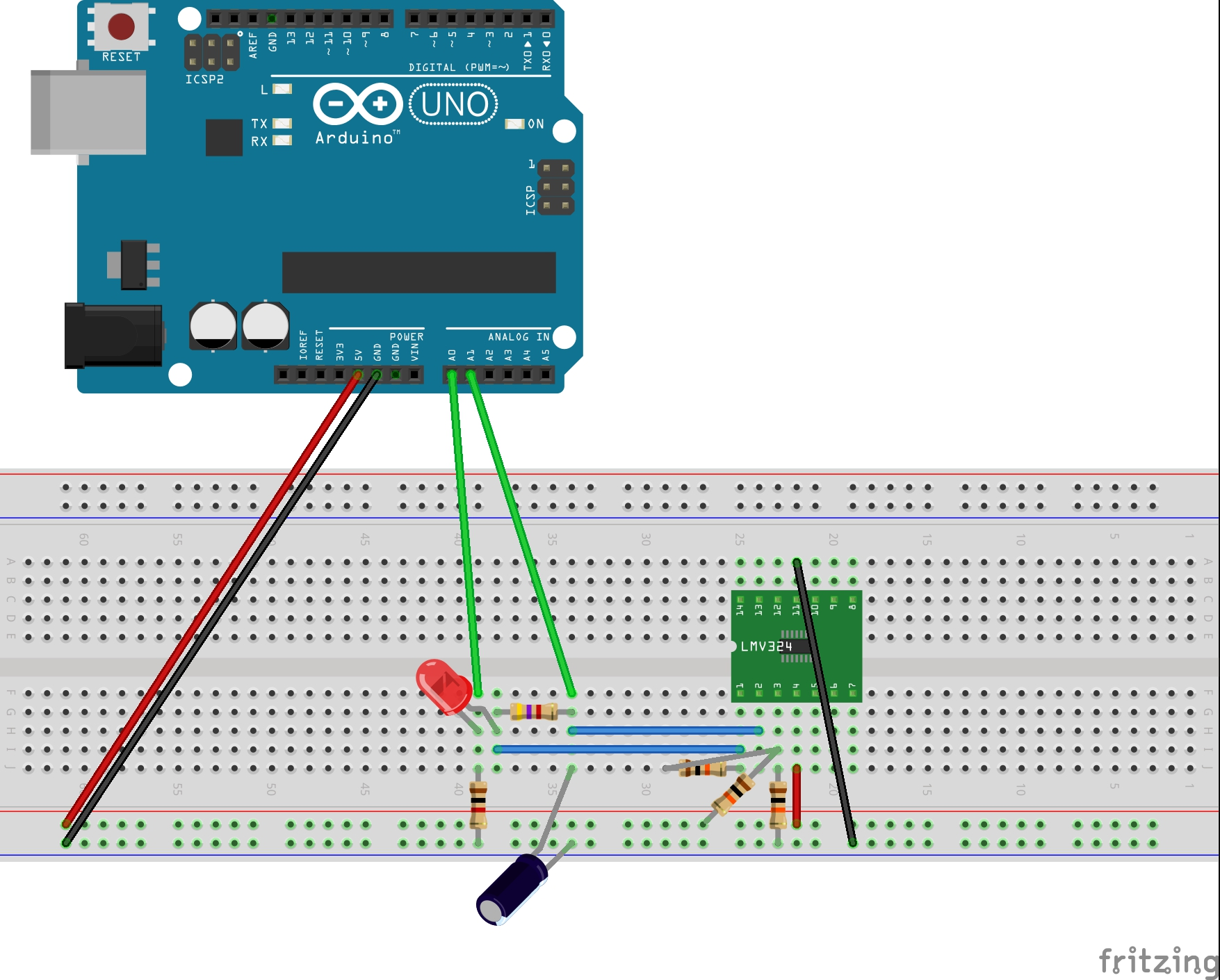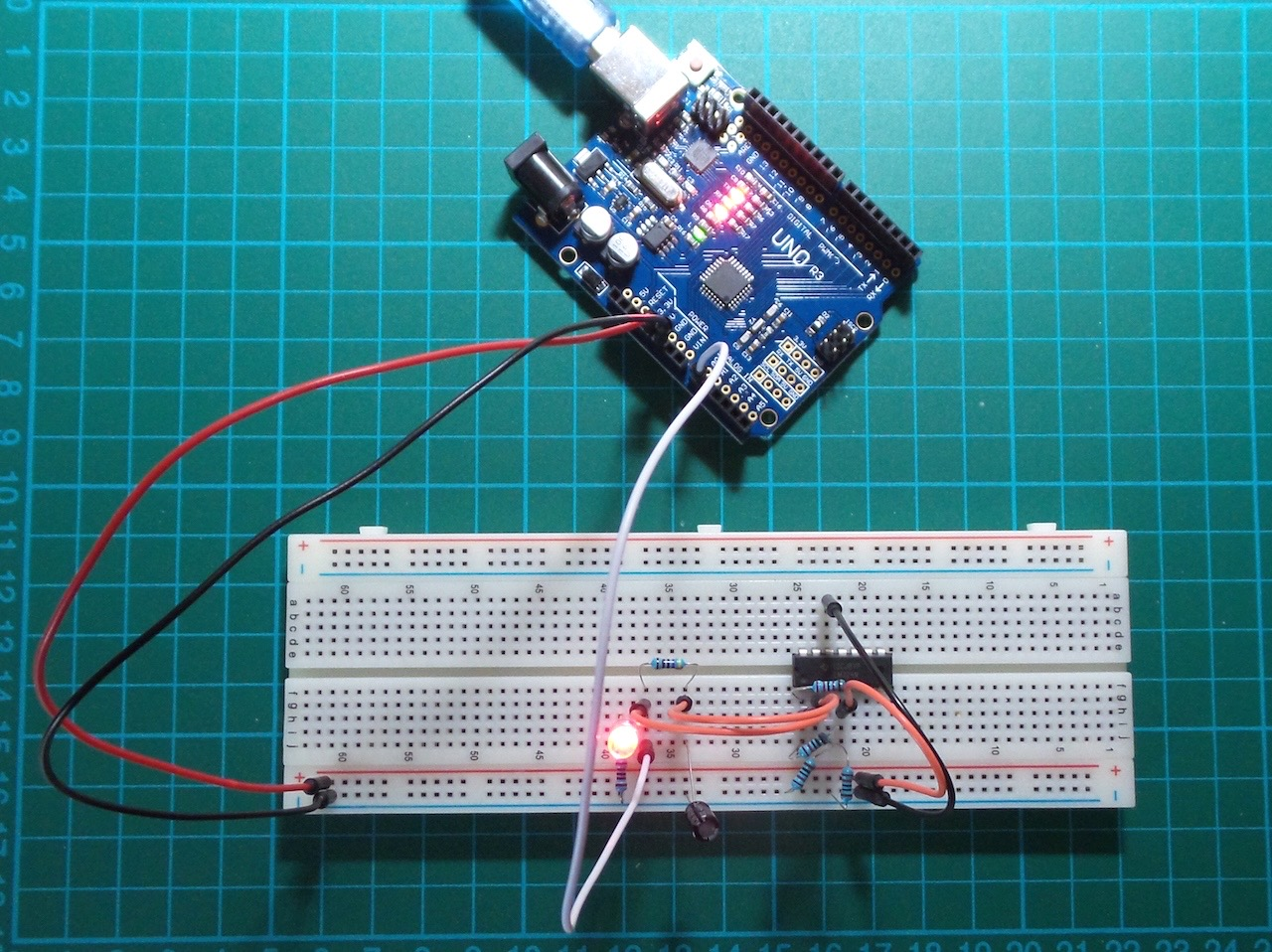# #039 Astable Opamp Oscillators

Use an Arduino to monitor the behaviour of an LM324 OpAmp astable oscillator and plot the results with Processing.

Demos the generation of a low-frequency square wave with an LM324 OpAmp.

The OpAmp unit is used as a comparator, with an RC circuit providing the oscillation.

The frequency is determined by the values of R4 and C1, and calculated using the formula:

``````frequency = 1/(2ln2 x RC)
``````

The values selected for the circuit yield a period of about 652ms, or 1.53Hz:

``````frequency = 1/(1.386 x 4700 x 0.0001)
frequency = 1.53Hz
``````

The square wave output is tapped at the base of the LED load. This ensures the “off” value gets fully pulled down to ground.

I’m also measuring the voltage at the inverted input. Interestingly, we get a reasonable triangle wave here, as its the middle of the RC network.

Here’s a sample trace of the oscillator output as measured by the Arduino. The lower trace is at the inverted input, the upper trace is the output.### Construction

The Arduino only acts as a measurement device in this circuit.

PlotNValues (a simple Processing sketch) reads the data from the serial port and plots the input and output value over time, with some coloration effects thrown in for good measure. In other words, we’re using Arduino and Processing as a basic oscilloscope! And it kind of works, mainly because the frequency is so low.NB: for simplicity, the circuit is powered from the Arduino 5V pin. That works fine, although it is equally possible to use a separate power supply (but ensuring there is a common ground connection).